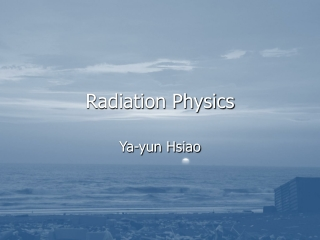DownloadDownload PresentationDownload Presentation- - - - - - - - - - - - - - - - - - - - - - - - - - - E N D - - - - - - - - - - - - - - - - - - - - - - - - - - -
##### Presentation Transcript

3. Example • A 6.2 mg sample of 90Sr (half-life 29.12 year) is in secular equilibrium with its daughter 90Y (half-life 64.0h). (a) how many Bq of 90Sr are present? (b) how many Bq of 90Y are present? (c) what is the mass of 90Y present? (d) what will the activity of 90Y be after 100 years?

4. Secular equilibrium: λSrNSr=λYNY NSr +NY =NTOTAL= NY= NSr=1.04x1016 atoms /3985.8=4.147x 1019 atoms A(t)= λN(t)

5. (a) • (b) • (c) • (d) mY=

6. Example • Consider the following beta decay chain with half-lives indicated, • 210Pb (22y) 210Bi (5d) 210Po • A sample contain 30 MBq of 210Pb and 15M Bq of 210Bi at t=0 (a) calculate the activity of 210Bi at time =10d (b) If the sample were originally pure 210Pb, how old would it have been at t=0?

7. Interactions of photons with matter • Photoelectric Effect • Compton Effect • Pair production

8. Photoelectric effect • Most of this energy goes to an atomic electron (with a small amount to the nucleus) resulting in a free electron and an ionized atom. • The photoelectric effect may only occur if the incident photon has an energy higher than the binding energy of the atomic electron.

9. Top insert to illustrate the interaction of a photon with an atom to eject an electron from the K shell to produce a photoelectron. When the hole in the K shell is filled, characteristic radiation is emitted. Main graph – Photoelectric attenuation coefficients for water and lead plotted on a log-log scale.

10. Compton Effect • An interaction between a photon and an atomic electron • An inelastic process • Some of the kinetic energy of the photon is required to overcome the binding energy of the atomic electron.

11. Compton Effect • And the recoil electron energy is given by • T = hν – hν' = • High energy incident photons suffer a large energy change, but low energy incident photons do not. This is important in radiotherapy since when the incident energy of the photon is large most of the available energy goes into kinetic energy of the recoil electron, which goes on to deposit energy in tissue, and very little energy goes to the scattered photon

12. Pair Production • Pair production is an interaction between a photon and a nuleus in which the photon is transformed into an electron‑positron pair. In the center of mass syst~m, the energy threshold for this e‑p pair creation is 2moC2 = 1.022 MeV.

13. Pair Production

14. Mass Attenuation Coefficient

15. Mass Energy Transfer Coefficient

16. Mass Energy Transfer Coefficient • Photons do not deposit energy in a material. It is the fast electrons that they produce following interaction that deposit energy. Over many interactions there will be an average energy transfer, , to the electron. • The mass energy transfer coefficient is tr/ρ

17. Mass Energy Absorption Coefficient Letting g represent the average fraction of the initial kinetic energy transferred to electrons that is subsequently emitted as bremsstrahlung

18. Mass Energy Absorption Coefficient